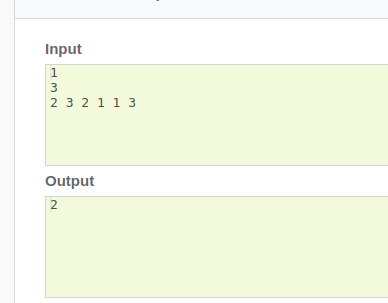# Getting a WA in CHCBOX, March-Cookoff 2020

I am getting a wrong answer with this solution.
Here’s my solution-
https://www.codechef.com/viewsolution/30669847
In the vector, I have replaced the non maximum values by 0 and the maximum values by 1.
‘bonus’ variable stores the required answer for all the consecutive 0’s in the vector except at the beginning and the end. ‘front’ and ‘end’ store the front and end 0’s respectively.

Thanks!the greedy approach is not right here

use prefix sum or suffix sum approach

@ajaymalik Isn’t the input format : input N and then N numbers, where N is even?
Here you have N=3(which is not even) and there are 6 numbers on the next line.

For test case
1
6
2 3 2 1 1 3
I’m getting 1 as the answer.your code is getting wa in this test case also

Shouldn’t the answer be 1 in this case?

by 4 right shift
arr = [2, 1, 1,3, 2 3] answer = 4 = > 4 shift
arr = [3, 2, 3, 2, 1, 1] answer = 8 = > 1 shift
4 < 8